Research papers and code for "Brendan O'Donoghue":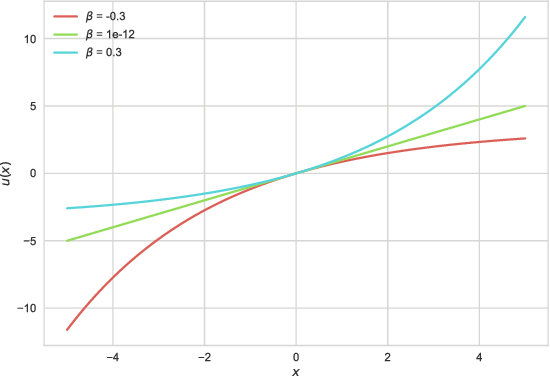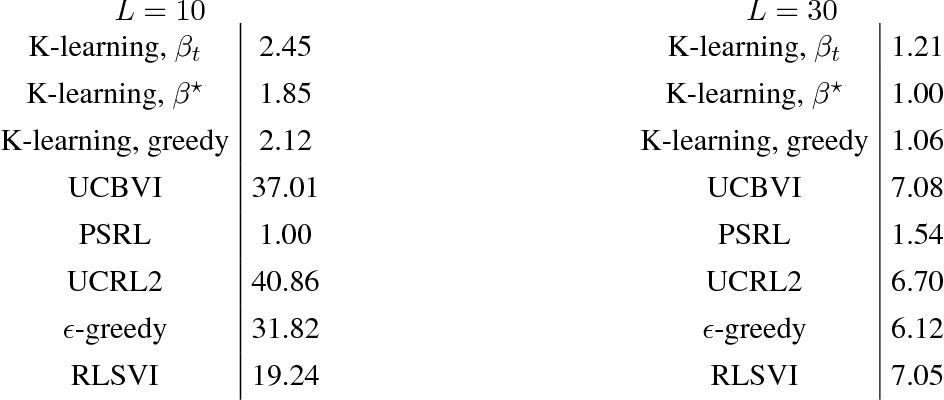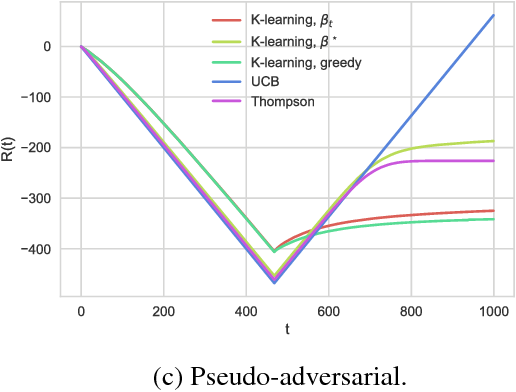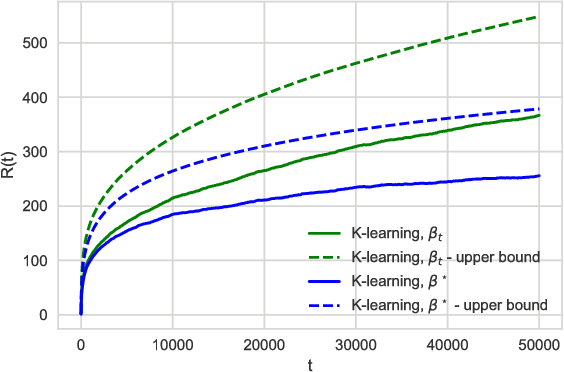We consider the exploration-exploitation trade-off in reinforcement learning and we show that an agent imbued with a risk-seeking utility function is able to explore efficiently, as measured by regret. The parameter that controls how risk-seeking the agent is can be optimized exactly, or annealed according to a schedule. We call the resulting algorithm K-learning and show that the corresponding K-values are optimistic for the expected Q-values at each state-action pair. The K-values induce a natural Boltzmann exploration policy for which the `temperature' parameter is equal to the risk-seeking parameter. This policy achieves an expected regret bound of $\tilde O(L^{3/2} \sqrt{S A T})$, where $L$ is the time horizon, $S$ is the number of states, $A$ is the number of actions, and $T$ is the total number of elapsed time-steps. This bound is only a factor of $L$ larger than the established lower bound. K-learning can be interpreted as mirror descent in the policy space, and it is similar to other well-known methods in the literature, including Q-learning, soft-Q-learning, and maximum entropy policy gradient, and is closely related to optimism and count based exploration methods. K-learning is simple to implement, as it only requires adding a bonus to the reward at each state-action and then solving a Bellman equation. We conclude with a numerical example demonstrating that K-learning is competitive with other state-of-the-art algorithms in practice.

Click to Read Paper and Get Code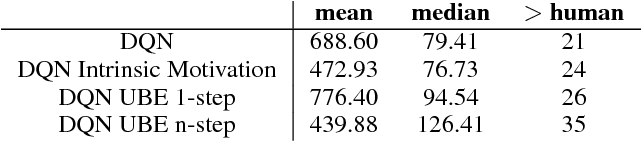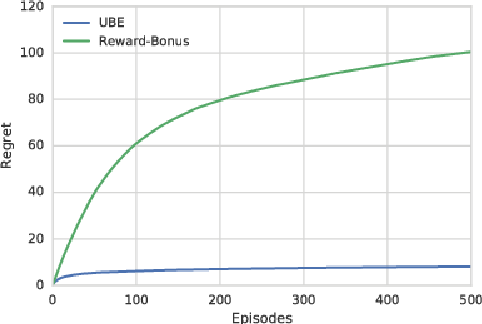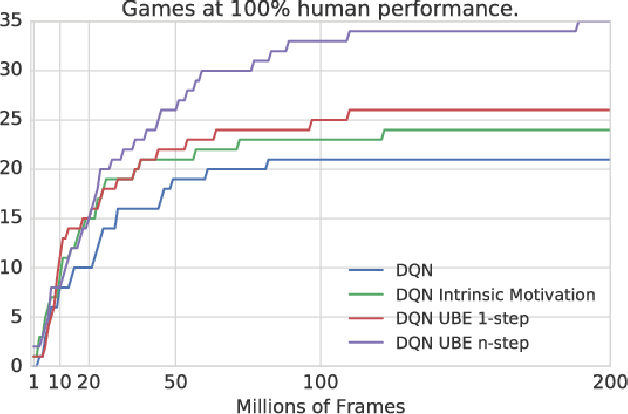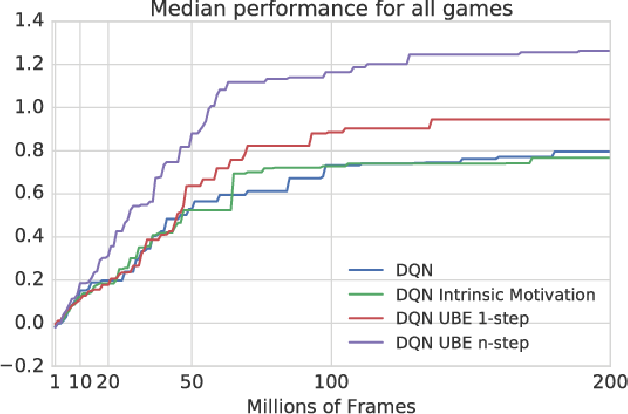We consider the exploration/exploitation problem in reinforcement learning. For exploitation, it is well known that the Bellman equation connects the value at any time-step to the expected value at subsequent time-steps. In this paper we consider a similar \textit{uncertainty} Bellman equation (UBE), which connects the uncertainty at any time-step to the expected uncertainties at subsequent time-steps, thereby extending the potential exploratory benefit of a policy beyond individual time-steps. We prove that the unique fixed point of the UBE yields an upper bound on the variance of the posterior distribution of the Q-values induced by any policy. This bound can be much tighter than traditional count-based bonuses that compound standard deviation rather than variance. Importantly, and unlike several existing approaches to optimism, this method scales naturally to large systems with complex generalization. Substituting our UBE-exploration strategy for $\epsilon$-greedy improves DQN performance on 51 out of 57 games in the Atari suite.

Click to Read Paper and Get Code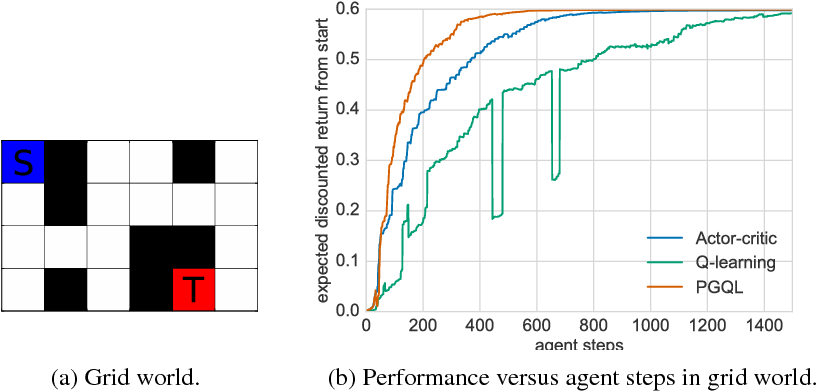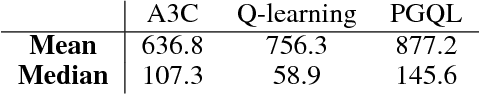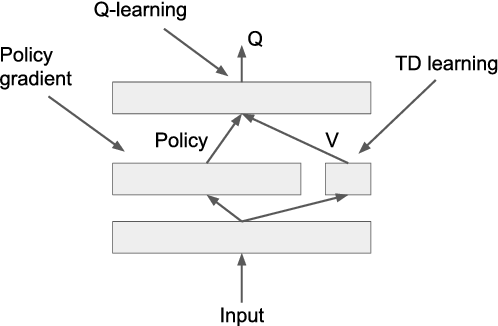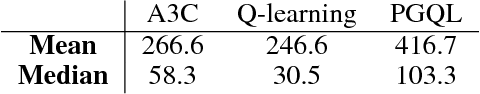Policy gradient is an efficient technique for improving a policy in a reinforcement learning setting. However, vanilla online variants are on-policy only and not able to take advantage of off-policy data. In this paper we describe a new technique that combines policy gradient with off-policy Q-learning, drawing experience from a replay buffer. This is motivated by making a connection between the fixed points of the regularized policy gradient algorithm and the Q-values. This connection allows us to estimate the Q-values from the action preferences of the policy, to which we apply Q-learning updates. We refer to the new technique as 'PGQL', for policy gradient and Q-learning. We also establish an equivalency between action-value fitting techniques and actor-critic algorithms, showing that regularized policy gradient techniques can be interpreted as advantage function learning algorithms. We conclude with some numerical examples that demonstrate improved data efficiency and stability of PGQL. In particular, we tested PGQL on the full suite of Atari games and achieved performance exceeding that of both asynchronous advantage actor-critic (A3C) and Q-learning.

Click to Read Paper and Get Code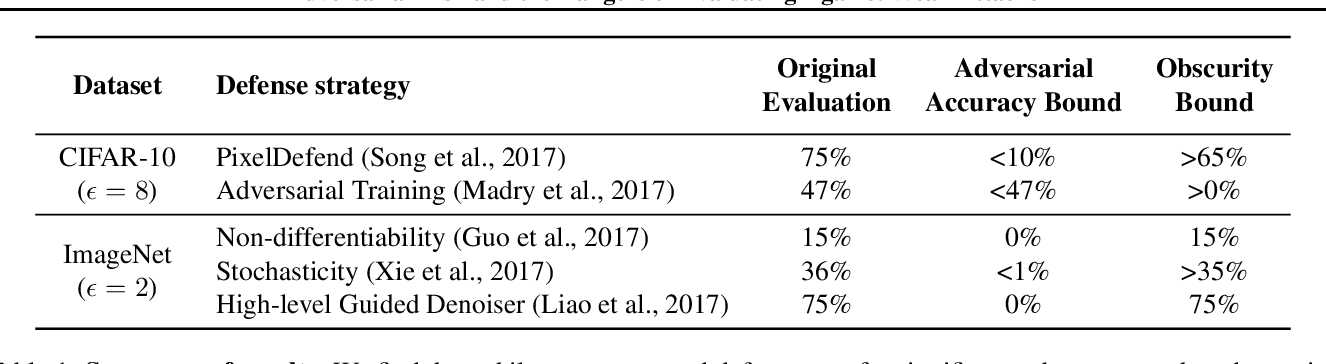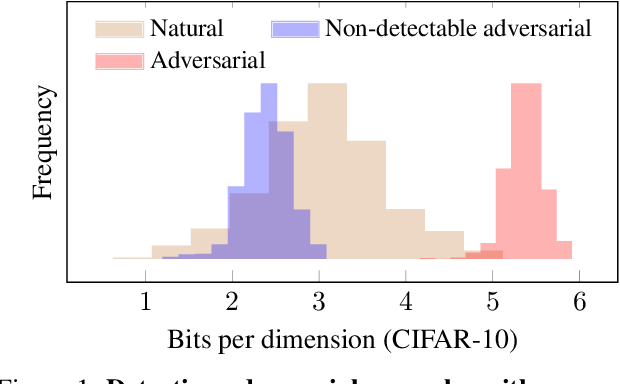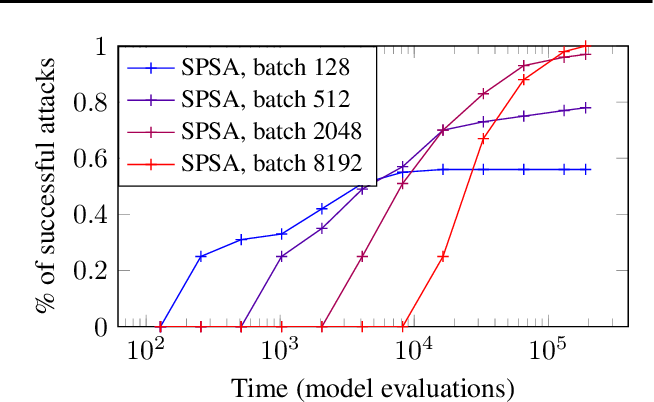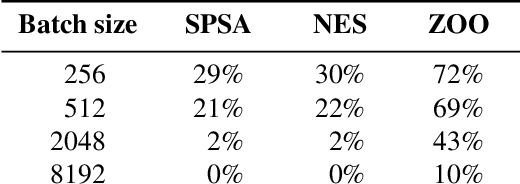This paper investigates recently proposed approaches for defending against adversarial examples and evaluating adversarial robustness. We motivate 'adversarial risk' as an objective for achieving models robust to worst-case inputs. We then frame commonly used attacks and evaluation metrics as defining a tractable surrogate objective to the true adversarial risk. This suggests that models may optimize this surrogate rather than the true adversarial risk. We formalize this notion as 'obscurity to an adversary,' and develop tools and heuristics for identifying obscured models and designing transparent models. We demonstrate that this is a significant problem in practice by repurposing gradient-free optimization techniques into adversarial attacks, which we use to decrease the accuracy of several recently proposed defenses to near zero. Our hope is that our formulations and results will help researchers to develop more powerful defenses.

Click to Read Paper and Get Code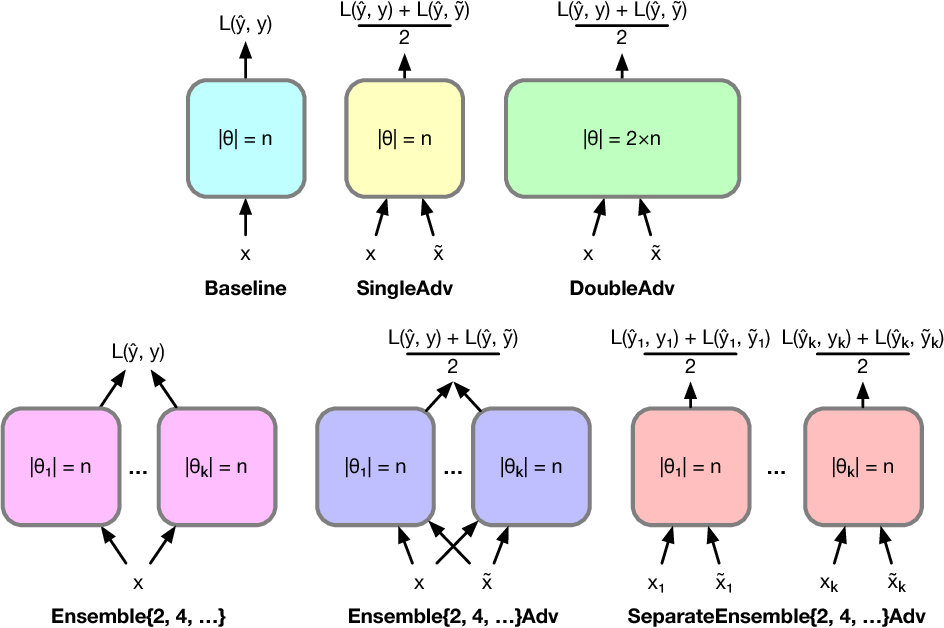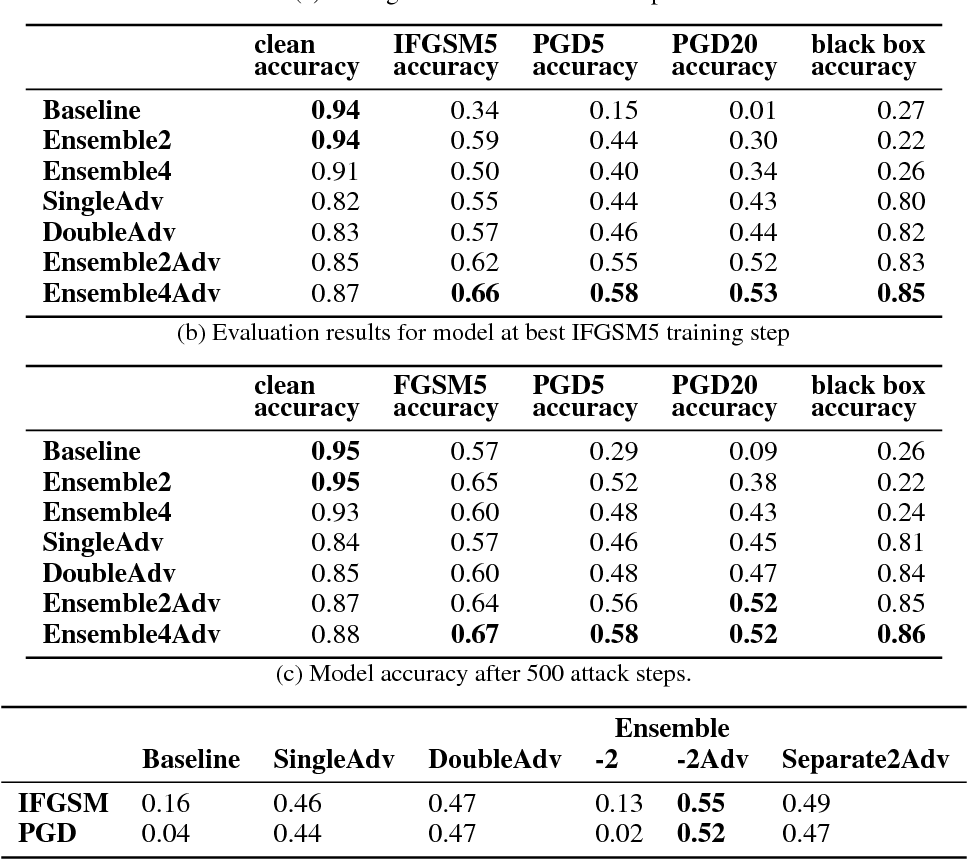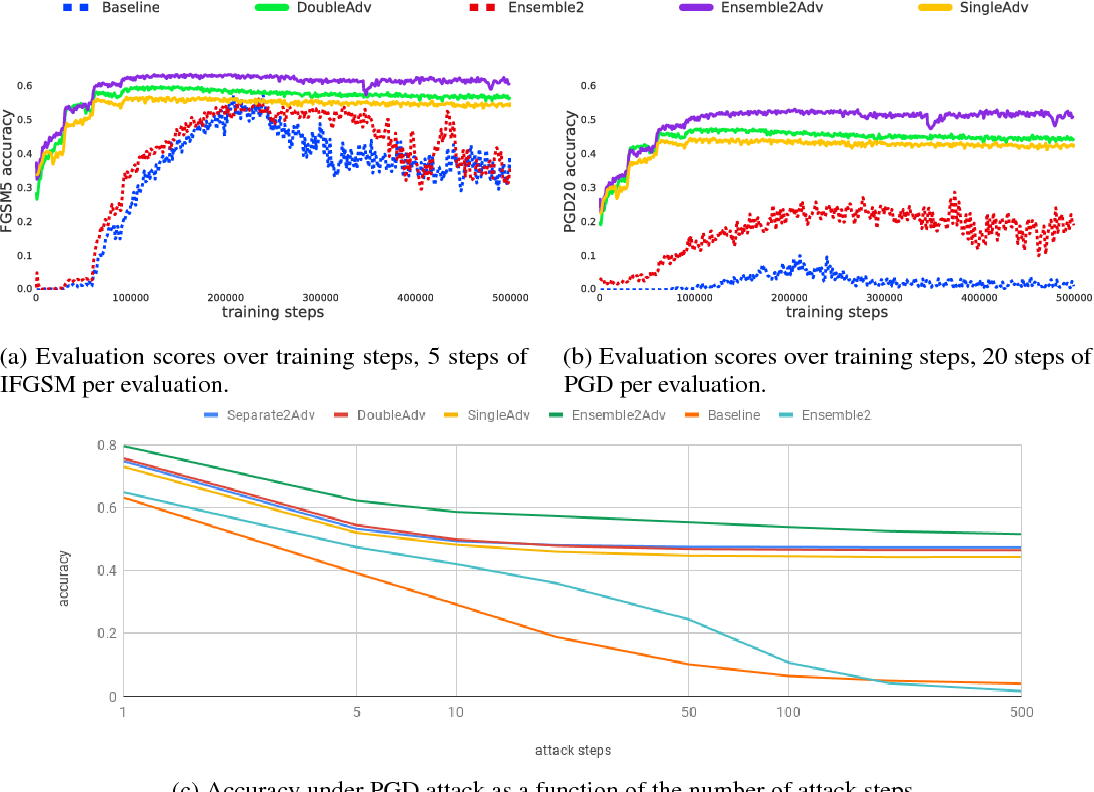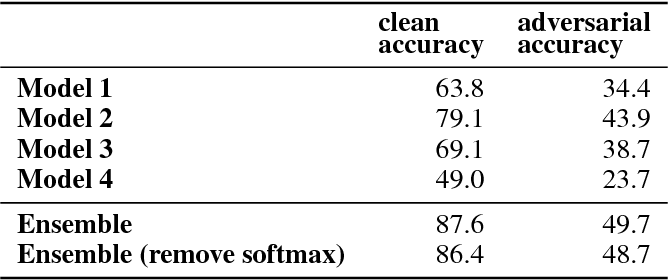While deep learning has led to remarkable results on a number of challenging problems, researchers have discovered a vulnerability of neural networks in adversarial settings, where small but carefully chosen perturbations to the input can make the models produce extremely inaccurate outputs. This makes these models particularly unsuitable for safety-critical application domains (e.g. self-driving cars) where robustness is extremely important. Recent work has shown that augmenting training with adversarially generated data provides some degree of robustness against test-time attacks. In this paper we investigate how this approach scales as we increase the computational budget given to the defender. We show that increasing the number of parameters in adversarially-trained models increases their robustness, and in particular that ensembling smaller models while adversarially training the entire ensemble as a single model is a more efficient way of spending said budget than simply using a larger single model. Crucially, we show that it is the adversarial training of the ensemble, rather than the ensembling of adversarially trained models, which provides robustness.

* 12 pages
Click to Read Paper and Get Code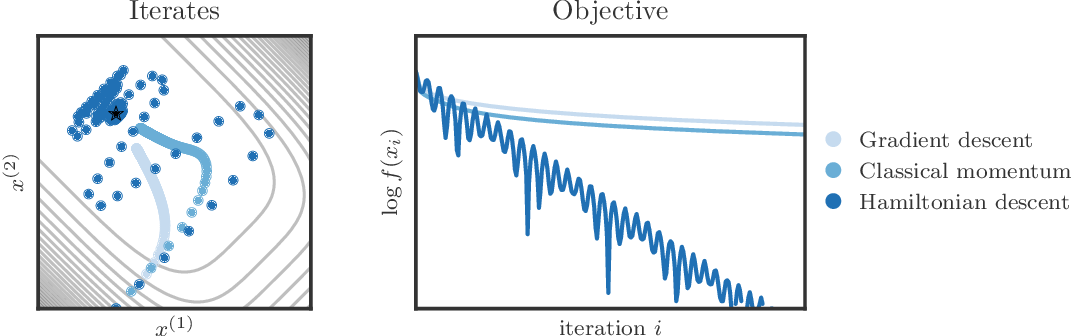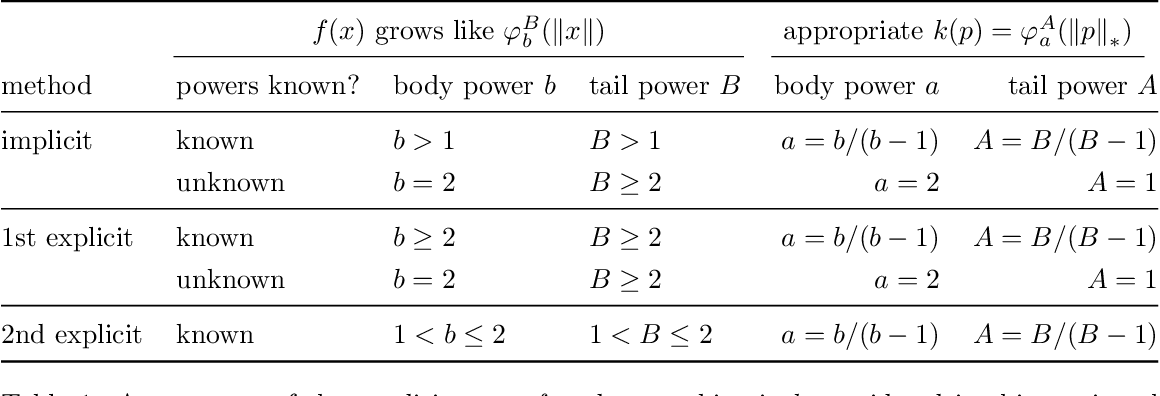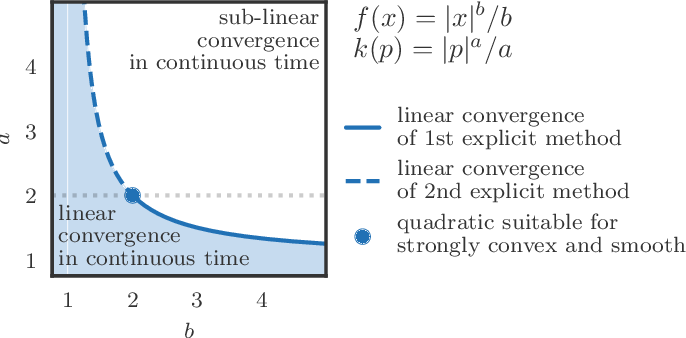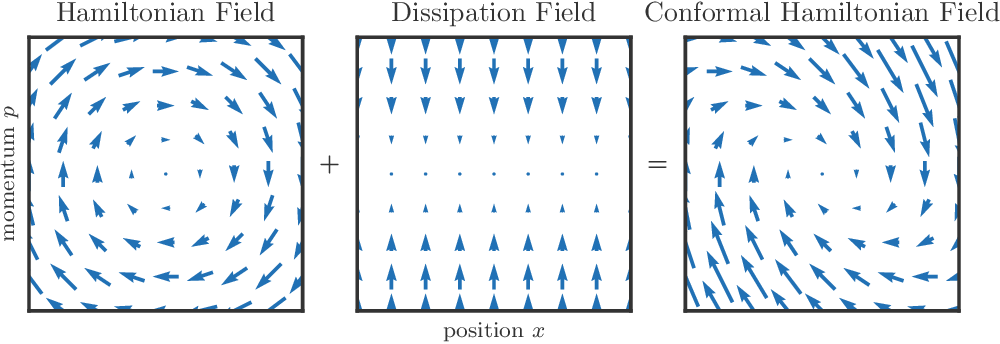We propose a family of optimization methods that achieve linear convergence using first-order gradient information and constant step sizes on a class of convex functions much larger than the smooth and strongly convex ones. This larger class includes functions whose second derivatives may be singular or unbounded at their minima. Our methods are discretizations of conformal Hamiltonian dynamics, which generalize the classical momentum method to model the motion of a particle with non-standard kinetic energy exposed to a dissipative force and the gradient field of the function of interest. They are first-order in the sense that they require only gradient computation. Yet, crucially the kinetic gradient map can be designed to incorporate information about the convex conjugate in a fashion that allows for linear convergence on convex functions that may be non-smooth or non-strongly convex. We study in detail one implicit and two explicit methods. For one explicit method, we provide conditions under which it converges to stationary points of non-convex functions. For all, we provide conditions on the convex function and kinetic energy pair that guarantee linear convergence, and show that these conditions can be satisfied by functions with power growth. In sum, these methods expand the class of convex functions on which linear convergence is possible with first-order computation.

Click to Read Paper and Get Code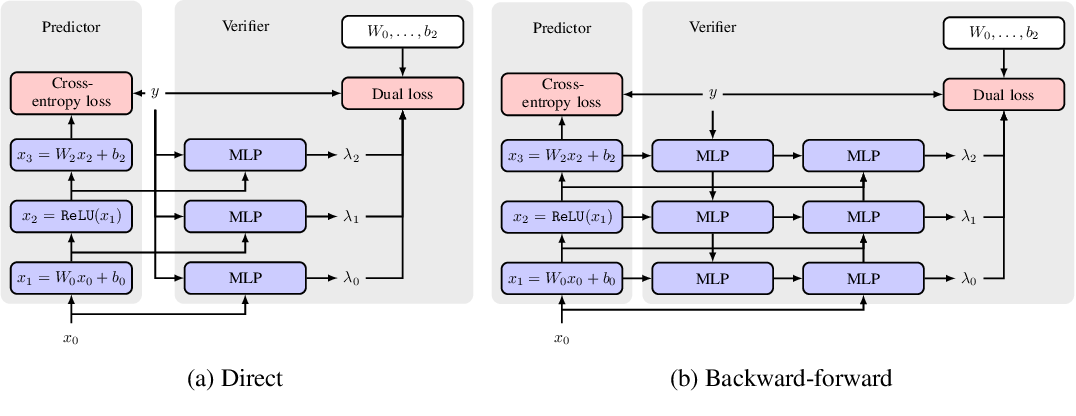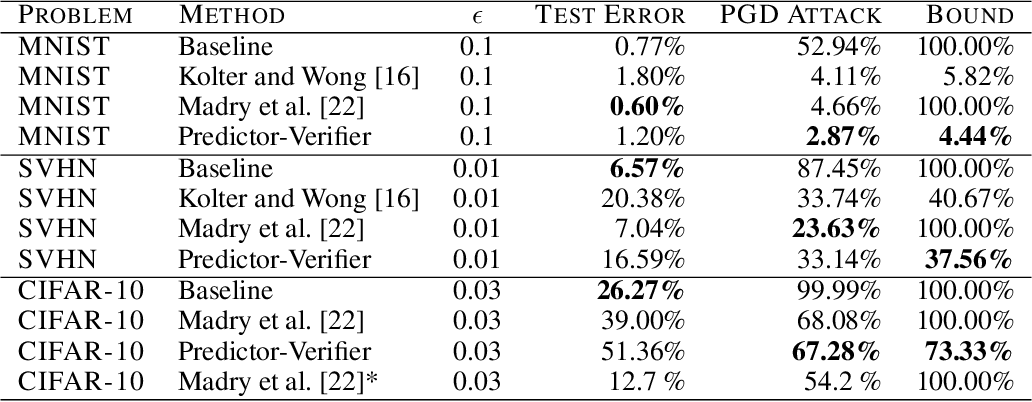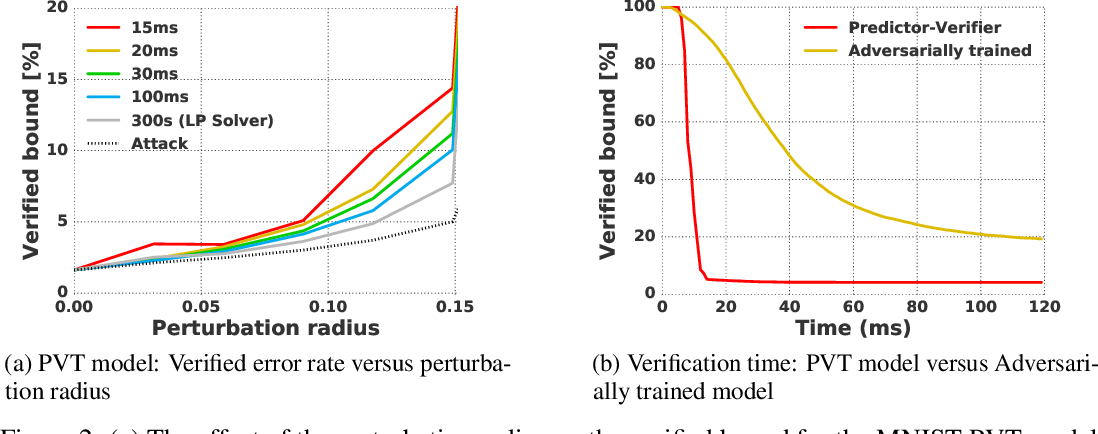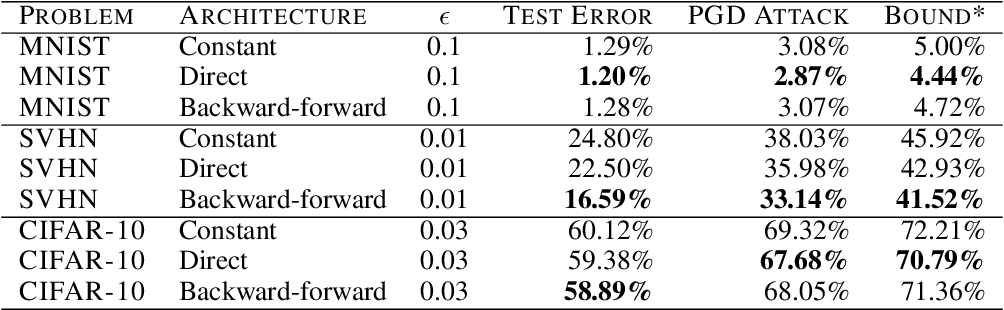This paper proposes a new algorithmic framework, predictor-verifier training, to train neural networks that are verifiable, i.e., networks that provably satisfy some desired input-output properties. The key idea is to simultaneously train two networks: a predictor network that performs the task at hand,e.g., predicting labels given inputs, and a verifier network that computes a bound on how well the predictor satisfies the properties being verified. Both networks can be trained simultaneously to optimize a weighted combination of the standard data-fitting loss and a term that bounds the maximum violation of the property. Experiments show that not only is the predictor-verifier architecture able to train networks to achieve state of the art verified robustness to adversarial examples with much shorter training times (outperforming previous algorithms on small datasets like MNIST and SVHN), but it can also be scaled to produce the first known (to the best of our knowledge) verifiably robust networks for CIFAR-10.

Click to Read Paper and Get Code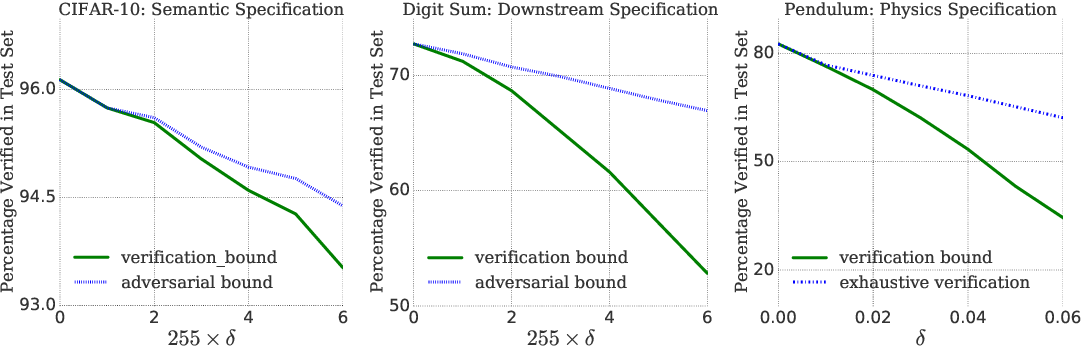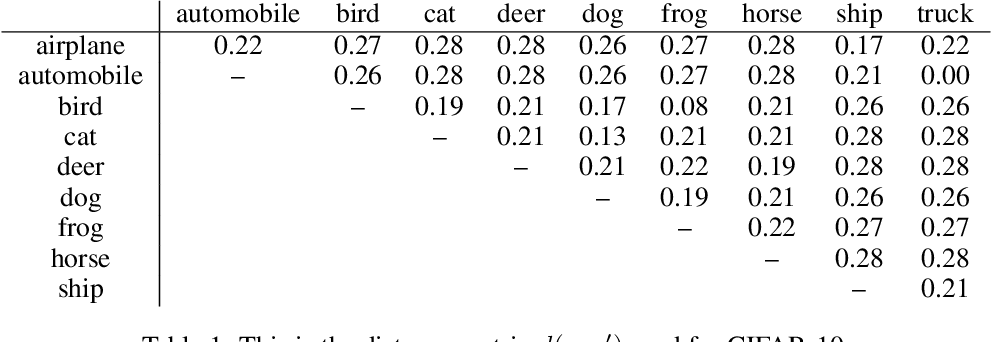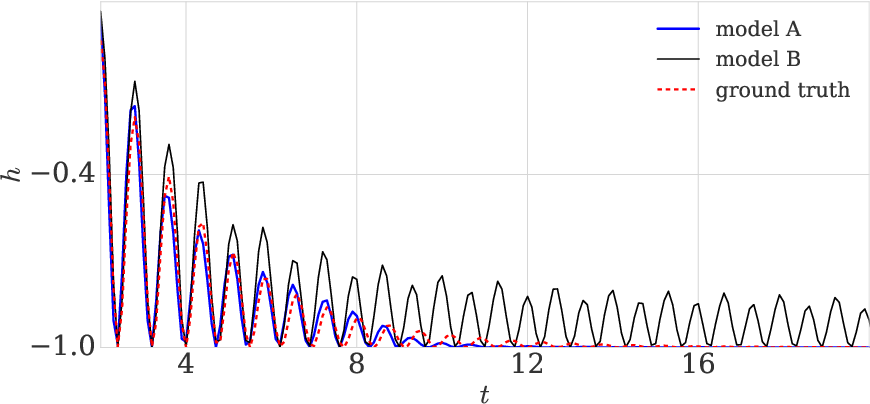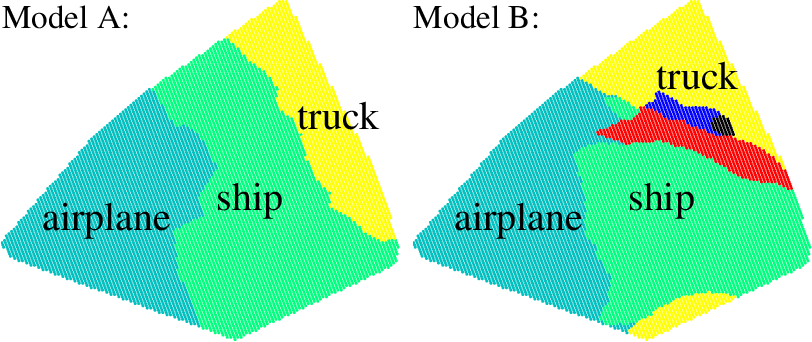Prior work on neural network verification has focused on specifications that are linear functions of the output of the network, e.g., invariance of the classifier output under adversarial perturbations of the input. In this paper, we extend verification algorithms to be able to certify richer properties of neural networks. To do this we introduce the class of convex-relaxable specifications, which constitute nonlinear specifications that can be verified using a convex relaxation. We show that a number of important properties of interest can be modeled within this class, including conservation of energy in a learned dynamics model of a physical system; semantic consistency of a classifier's output labels under adversarial perturbations and bounding errors in a system that predicts the summation of handwritten digits. Our experimental evaluation shows that our method is able to effectively verify these specifications. Moreover, our evaluation exposes the failure modes in models which cannot be verified to satisfy these specifications. Thus, emphasizing the importance of training models not just to fit training data but also to be consistent with specifications.

* ICLR conference paper
Click to Read Paper and Get Code